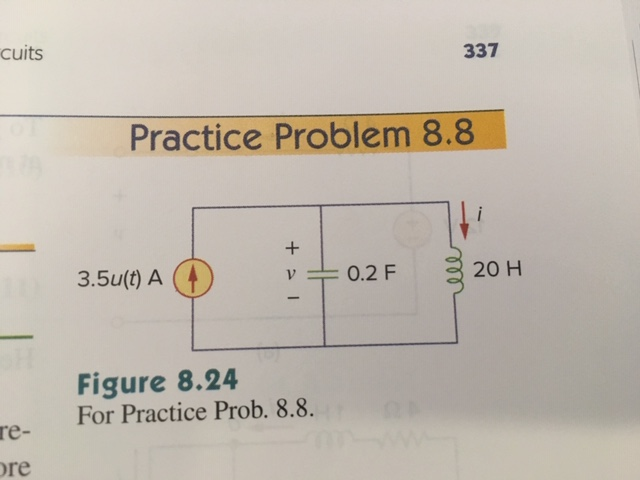# cuits337Practice Problem 8.83.5ut)A0.2F20 HFigure 8.24For Practice Prob. 8.8re-re

Question
371 views

Find i(t) and v(t) for t >0 in the circuit in the figure attached.help_outlineImage Transcriptionclosecuits 337 Practice Problem 8.8 3.5ut)A 0.2F 20 H Figure 8.24 For Practice Prob. 8.8 re- re fullscreen
check_circle

Step 1

Write the general expression for unit step function,

Step 2

Figure 1 looks like a parallel  circuit with the value of resistance is equal to infinity. The value of source current (Is) is 3.5 A. Therefore, the neper frequency for parallel  circuit is calculated as follows.

Step 3

On comparing the neper and natural frequency, the value o...

### Want to see the full answer?

See Solution

#### Want to see this answer and more?

Solutions are written by subject experts who are available 24/7. Questions are typically answered within 1 hour.*

See Solution
*Response times may vary by subject and question.
Tagged in